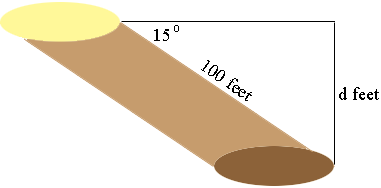SEARCH HOMEMath Central Quandaries & QueriesQuestion from Scott: If I have a hole three foot in circumference that runs at a 15degree angle from the surface and continues for a length of 100 feet, how deep would the hole be at 100 feet?Hi Scott,

You are looking for d in my diagramThe tangent of an angle in a right triangle is the length of the side opposite the angle divided by the length of the hypotenuse. In your situation this is

sin(15o) = d/100

and thus

d = 100 sin(15o)

Use your calculator to find 15o (make sure it is in degree mode) and then multiply by 100.

PennyMath Central is supported by the University of Regina and The Pacific Institute for the Mathematical Sciences.